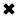# What is Voltage?

#### By R. W. Hurst, President, The Electricity Forum

What is Voltage?

Voltage is the standard unit of electromotive force (EMF) or potential difference, measured in volts. Voltage is an accumulation of static electric charge flowing in one direction, such as an excess or shortage of electrons measured, is always, associated with voltage. There are other situations in which voltage exists. Voltage is generated at a power plant, and produced in an electrochemical reaction, and caused by light falling on a special semiconductor chip. It can be produced when an object is moved in a magnetic field, or is placed in a fluctuating magnetic field.

An electric potential difference between two points produces an electric field, represented by electric lines of flux (Fig. 1). There is always a pole that is relatively positive, with fewer electrons, and one that is relatively negative, with more electrons. The positive pole does not necessarily have a deficiency of electrons compared with neutral objects, and the negative pole might not actually have a surplus of electrons with respect to neutral things. But there's always a difference in charge between the two poles. The negative pole always has more electrons than the positive pole.Fig 1. Electric lines of flux always exist near poles of electric charge.

The abbreviation for voltage is V. Sometimes, smaller units are used. The millivolt (mV) is equal to a thousandth (0.001) of a volt. The microvolt (uV) is equal to a millionth (0.000001) of a volt. And it is sometimes necessary to use units much larger than one volt. One kilovolt (kV) is equal to one thousand volts (1,000). One megavolt (MV) is equal to one million volts (1,000,000) or one thousand kilovolts.

In a dry cell, the EMF is usually between 1.2 and 1.5 volts; in a car battery, it is most often 12 V to 14 V. In household utility wiring, it is a low-frequency alternating current of about 117 V for electric lights and most appliances, and 234 V for a washing machine, dryer, oven, or stove. In television sets, transformers convert 117 V to around 450 V for the operation of the picture tube. In some broadcast transmitters, kilovolts are used. The largest voltages on Earth occur between clouds, or between clouds and the ground, in thundershowers; this potential difference is on the order of tens of megavolts.

In every case, measuring voltage electrical, EMF, or potential difference represents the fact that current flows between two points if a conductive path is provided. The number of charge carriers might be small even if the voltage is huge, or very large even if the voltage is tiny. Voltage dropped represents the pressure or driving force that impels the charge to move. In general, for a given number of charge carriers, higher voltages will produce a faster flow, and therefore a larger current. It's something like water pressure. The amount of water that will flow through a hose is proportional to the water pressure, all other things being equal.

A potential difference between two points, called poles, invariably produces an electric field, represented by electric lines offiux as shown in Fig.1. We call such a pair of electric charge poles an electric dipole. One pole carries a relatively positive charge, and the other pole carries a relatively negative charge. The positive pole always has fewer electrons than the negative pole. Note that the electron numbers are relative, not absolute! An electric dipole can exist even if both poles carry surplus electrons, or if both poles suffer from electron deficiencies, relative to some external point of reference having an absolutely neutral charge.

The abbreviation for voltage (or volts) is V. Sometimes, engineers use smaller units. The millivolt (mV) equals 0.001 V. The microvolt (tV) equals 0.000001 V. Units larger than the volt also exist. One kilovolt (kV) represents 1000 V. One megavolt (MV) equals 1,000,000 V or 1000 kV. This is related to ohm law.

In an everyday dry cell, the poles maintain a potential difference somewhere between 1.2 and 1-7 V. In an automotive battery, it's in the range of 12 V to 14 V. In household AC utility wiring, the potential difference alternates polarity and maintains an effective value of approximately 117 V. Electric lights and most small appliances, and 234 V for washing machines, ovens, or other large appliances. In some high-power radio transmitters, the EMF can range in the thousands of volts. The largest potential differences on our planet—upwards of 1 MV—build up in thunderstorms, sandstorms, and erupting volcanoes.

To understand what is voltage, voltage always means that charge carriers, which are mostly electrons in a conventional electrical circuit, will travel between the charged poles if we provide a decent path for them to follow. Voltage represents the driving force, or "pressure," that impels charge carriers to move. If we hold all other factors constant, a high voltage will make the charge carriers flow in greater quantity per unit time, thereby producing a larger electrical current, than a low voltage. But that statement oversimplifies the situation in most practical systems, where "all other factors" rarely "hold constant"!

So, what is voltage? Voltage is the difference in electric potential between two points, which (in a static electric field) is defined as the work needed per unit of charge to move a test charge between the two points.

##### Basic Electricity Handbook, Vol. 1• FULLY ILLUSTRATED
• GREAT FOR SCHOOL PROJECTS
• GREAT PRICE: \$5.99
• ...

This 100+ page e-book is a great guide for those who have a basic interest in the field of electricity. This well-illustrated e-book, coupled with some basic knowledge of electricity, will give you a broad theoretical background in this fundamental subject.

CONTENTS
• FUNDAMENTALS OF ELECTRICITY
• HOW DOES ELECTRICITY WORK?
• HOW IS ELECTRICITY GENERATED?
• WHERE DOES ELECTRICITY COME FROM?
• BASIC HOME WIRING
• ELECTRICITY FOR STUDENTS
• ENERGY SAVING TIPS

##### TRAINING EF COURSES

Content Community Connection
Top